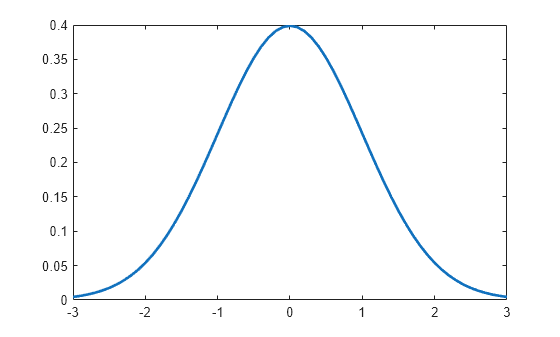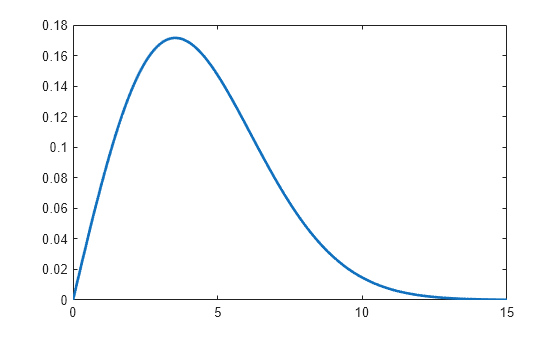# pdf

## 语法

``y = pdf(name,x,A)``
``y = pdf(name,x,A,B)``
``y = pdf(name,x,A,B,C)``
``y = pdf(name,x,A,B,C,D)``
``y = pdf(pd,x)``

## 说明

``y = pdf(name,x,A)` 返回由 `name` 和分布参数 `A` 指定的单参数分布族的概率密度函数 (pdf)，在 `x` 中的值处计算函数值。`

``y = pdf(name,x,A,B)` 返回由 `name` 以及分布参数 `A` 和 `B` 指定的双参数分布族的 pdf，在 `x` 中的值处计算函数值。`
``y = pdf(name,x,A,B,C)` 返回由 `name` 以及分布参数 `A`、`B` 和 `C` 指定的三参数分布族的 pdf，在 `x` 中的值处计算函数值。`
``y = pdf(name,x,A,B,C,D)` 返回由 `name` 以及分布参数 `A`、`B`、`C` 和 `D` 指定的四参数分布族的 pdf，在 `x` 中的值处计算函数值。`

``y = pdf(pd,x)` 返回概率分布对象 `pd` 的 pdf，在 `x` 中的值处计算函数值。`

## 示例

`x = [-2 -1 0 1 2];`

```mu = 1; sigma = 5; y = pdf('Normal',x,mu,sigma)```
```y = 1×5 0.0666 0.0737 0.0782 0.0798 0.0782 ```

`y` 中的每个值对应于输入向量 `x` 中的一个值。例如，在值 `x` 等于 1 处，`y` 中对应的 pdf 值等于 0.0798。

```mu = 1; sigma = 5; pd = makedist('Normal','mu',mu,'sigma',sigma);```

`x = [-2 -1 0 1 2];`

`y = pdf(pd,x)`
```y = 1×5 0.0666 0.0737 0.0782 0.0798 0.0782 ```

`y` 中的每个值对应于输入向量 `x` 中的一个值。例如，在值 `x` 等于 1 处，`y` 中对应的 pdf 值等于 0.0798。

```lambda = 2; pd = makedist('Poisson','lambda',lambda);```

`x = [0 1 2 3 4];`

`y = pdf(pd,x)`
```y = 1×5 0.1353 0.2707 0.2707 0.1804 0.0902 ```

y 中的每个值对应于输入向量 x 中的一个值。例如，在值 x 等于 3 处，y 中对应的 pdf 值等于 0.1804。

`y2 = pdf('Poisson',x,lambda)`
```y2 = 1×5 0.1353 0.2707 0.2707 0.1804 0.0902 ```

pdf 值与使用概率分布对象计算的值相同。

`pd = makedist('Normal')`
```pd = NormalDistribution Normal distribution mu = 0 sigma = 1 ```

```x = -3:.1:3; pdf_normal = pdf(pd,x);```

`plot(x,pdf_normal,'LineWidth',2)``pd = makedist('Weibull','A',5,'B',2)`
```pd = WeibullDistribution Weibull distribution A = 5 B = 2 ```

```x = 0:.1:15; y = pdf(pd,x);```

`plot(x,y,'LineWidth',2)`## 输入参数

`name`分布输入参数 `A`输入参数 `B`输入参数 `C`输入参数 `D`
`'Beta'`Beta Distributiona 第一个形状参数b 第二个形状参数不适用不适用
`'Binomial'`Binomial Distributionn 试验次数p 每次试验成功的概率不适用不适用
`'BirnbaumSaunders'`Birnbaum-Saunders Distributionβ 尺度参数γ 形状参数不适用不适用
`'Burr'`Burr Type XII Distributionα 尺度参数c 第一个形状参数k 第二个形状参数不适用
`'Chisquare'``'chi2'`Chi-Square Distributionν 自由度不适用不适用不适用
`'Exponential'`Exponential Distributionμ 均值不适用不适用不适用
`'Extreme Value'``'ev'`Extreme Value Distributionμ 位置参数σ 尺度参数不适用不适用
`'F'`F Distributionν1 分子自由度ν2 分母自由度不适用不适用
`'Gamma'`Gamma Distributiona 形状参数b 尺度参数不适用不适用
`'Generalized Extreme Value'``'gev'`Generalized Extreme Value Distributionk 形状参数σ 尺度参数μ 位置参数不适用
`'Generalized Pareto'``'gp'`Generalized Pareto Distributionk 尾部指数（形状）参数σ 尺度参数μ 阈值（位置）参数不适用
`'Geometric'`Geometric Distributionp 概率参数不适用不适用不适用
`'Half Normal'``'hn'`Half-Normal Distributionμ 位置参数σ 尺度参数不适用不适用
`'Hypergeometric'``'hyge'`Hypergeometric Distributionm 总体的大小k 总体中具有所需特征的项数n 抽取的样本数量不适用
`'InverseGaussian'`Inverse Gaussian Distributionμ 尺度参数λ 形状参数不适用不适用
`'Logistic'`逻辑分布μ 均值σ 尺度参数不适用不适用
`'LogLogistic'`Loglogistic Distributionμ 对数值的均值σ 对数值的尺度参数不适用不适用
`'LogNormal'`对数正态分布μ 对数值的均值σ 对数值的标准差不适用不适用
`'Loguniform'`Loguniform Distributiona 下部端点（最小值）b 上部端点（最大值）不适用不适用
`'Nakagami'`Nakagami Distributionμ 形状参数ω 尺度参数不适用不适用
`'Negative Binomial'``'nbin'`Negative Binomial Distributionr 成功次数p 单个试验的成功概率不适用不适用
`'Noncentral F'``'ncf'`Noncentral F Distributionν1 分子自由度ν2 分母自由度δ 非中心参数不适用
`'Noncentral t'``'nct'`Noncentral t Distributionν 自由度δ 非中心参数不适用不适用
`'Noncentral Chi-square'``'ncx2'`Noncentral Chi-Square Distributionν 自由度δ 非中心参数不适用不适用
`'Normal'`正态分布μ 均值 σ 标准差不适用不适用
`'Poisson'`泊松分布λ 均值不适用不适用不适用
`'Rayleigh'`瑞利分布b 尺度参数不适用不适用不适用
`'Rician'`Rician Distributions 非中心参数σ 尺度参数不适用不适用
`'Stable'`Stable Distributionα 第一个形状参数β 第二个形状参数γ 尺度参数δ 位置参数
`'T'`Student's t Distributionν 自由度不适用不适用不适用
`'tLocationScale'`t Location-Scale Distributionμ 位置参数σ 尺度参数ν 形状参数不适用
`'Uniform'`Uniform Distribution (Continuous)a 下部端点（最小值）b 上部端点（最大值）不适用不适用
`'Discrete Uniform'``'unid'`Uniform Distribution (Discrete)n 最大可观测值不适用不适用不适用
`'Weibull'``'wbl'`Weibull Distributiona 尺度参数b 形状参数不适用不适用

`BetaDistribution``makedist``fitdist`分布拟合器
`BinomialDistribution``makedist``fitdist`分布拟合器
`BirnbaumSaundersDistribution``makedist``fitdist`分布拟合器
`BurrDistribution``makedist``fitdist`分布拟合器
`ExponentialDistribution``makedist``fitdist`分布拟合器
`ExtremeValueDistribution``makedist``fitdist`分布拟合器
`GammaDistribution``makedist``fitdist`分布拟合器
`GeneralizedExtremeValueDistribution``makedist``fitdist`分布拟合器
`GeneralizedParetoDistribution``makedist``fitdist`分布拟合器
`HalfNormalDistribution``makedist``fitdist`分布拟合器
`InverseGaussianDistribution``makedist``fitdist`分布拟合器
`KernelDistribution``fitdist`分布拟合器
`LogisticDistribution``makedist``fitdist`分布拟合器
`LoglogisticDistribution``makedist``fitdist`分布拟合器
`LognormalDistribution``makedist``fitdist`分布拟合器
`LoguniformDistribution``makedist`
`MultinomialDistribution``makedist`
`NakagamiDistribution``makedist``fitdist`分布拟合器
`NegativeBinomialDistribution``makedist``fitdist`分布拟合器
`NormalDistribution``makedist``fitdist`分布拟合器

`PiecewiseLinearDistribution``makedist`
`PoissonDistribution``makedist``fitdist`分布拟合器
`RayleighDistribution``makedist``fitdist`分布拟合器
`RicianDistribution``makedist``fitdist`分布拟合器
`StableDistribution``makedist``fitdist`分布拟合器
`tLocationScaleDistribution``makedist``fitdist`分布拟合器
`TriangularDistribution``makedist`
`UniformDistribution``makedist`
`WeibullDistribution``makedist``fitdist`分布拟合器

## 输出参数

pdf 值，以标量值或标量值数组的形式返回。在经过任何必要的标量扩展后，`y` 的大小与 `x` 相同。`y` 中的每个元素均为由分布参数（`A``B``C``D`）中的对应元素指定或由概率分布对象 (`pd`) 指定的分布的 pdf 值，其值在 `x` 中的对应元素处进行计算。